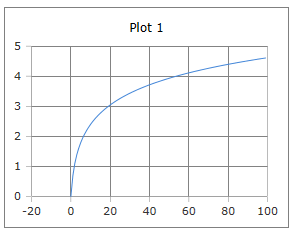# Ln, logarithm to base e

Calculator and formula for calculating the logarithm for base e

## Calculate the logarithm of the base e

The function Ln returns the logarithm to the base e of the specified number (power value). A real number must be given as an argument. The Ln function for complex numbers can be found here

To perform the calculation, enter a real number and then click the 'Calculate' button.

Calculator of logarithm to base e

 Input Power value Decimal places 0 1 2 3 4 6 8 10 Result Ln## Formula for the logarithm for base e

$$\displaystyle ln(x)= log_{e}(x)=\frac{log\,(x)}{log\,(e)}=y$$